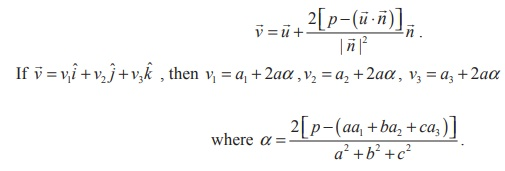Home | | Maths 12th Std | Image of a Point in a Plane

# Image of a Point in a Plane

The coordinates of the image of a point in a plane

Image of a Point in a Plane

Let A be the given point whose position vector is. Let= p be the equation of the plane.

Letbe the position vector of the mirror image A′ of A in the plane. Thenis perpendicular to the plane. So it is parallel to. ThenLet M be the middle point of AA′. Then the position vector of M is. But M lies on the plane.### Note

The mid point of M of AA′ is the foot of the perpendicular from the point A to the plane.p.

So the position vector of the foot M of the perpendicular is given by .## The coordinates of the image of a point in a plane

Let (a1, a2, a 3) be the pointwhose image in the plane is required. Thena1ˆi a2ˆj a3ˆk

Let ax + by + cz = d be the equation of the given plane. Writing the equation in the vector form we get.p where= aˆi + bˆj + cˆk Then the position vector of the image is### Example 6.55

Find the image of the point whose position vector is ˆi + 2ˆj + 3ˆk in the plane(ˆi + 2ˆj + 4ˆk ) = 38 .

### SolutionTherefore, the image of the point with position vector iˆ + 2 ˆj + 3kˆ is 2iˆ + 4 ˆj + 7kˆ .

Note

The foot of the perpendicular from the point with position vector iˆ + 2ˆj + 3kˆ in the given plane isStudy Material, Lecturing Notes, Assignment, Reference, Wiki description explanation, brief detail
12th Mathematics : UNIT 6 : Applications of Vector Algebra : Image of a Point in a Plane |